# SilverZone - iRAO PDF Sample Papers for Class 6

Resources:

Class 6 sample paper & practice questions for International Reasoning & Aptitude Olympiad (iRAO) level 1 are given below. Syllabus for level 1 is also mentioned for these exams. You can refer these sample paper & quiz for preparing for the exam.Q.1 Q.2 Q.3 Q.4 Q.5 Q.6 Q.7 Q.8 Q.9 Q.10
 Q.1 Given: A denotes Addition, B denotes Subtraction, C denotes Multiplication, D denotes Division, E denotes equal to, F denotes Greater than, G denotes less than. Answer the following question accordingly. Choose the correct equation out of these. a) 3 A 4 F 12 b) 3 C 4 E 12 c) 3 A 4 E 12 d) 3 C 4 G 12
 Q.2 Find out the missing term indicated by '?' JKL: LMN::?: NOP a) JMO b) STU c) PQR d) LMN
 Q.3 In the following question, find out which of the answer figures (1), (2), (3) and (4) completes the figure matrix?Select a suitable figure from the four alternatives that would complete the figure matrix.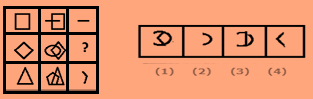a) 2 b) 1 c) 4 d) 3
 Q.4 Choose a figure which would most closely resemble the unfolded form of Figure (Z).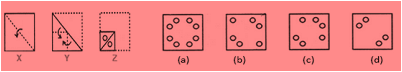a) a b) b c) c d) d
 Q.5 Insert a rational number between 3/2 and 5/2 a) 2 b) 0 c) 1 d) 4
 Q.6 Which of these is a cone? a)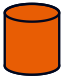b)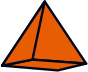c)d)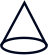Q.7 On a playing ground Sachin, Balu, Kiran, Kasi and Puja are standing as described below facing North: 1. Balu is 40 m to East of Kasi. 2. Sachin is 60 m to the South of Balu. 3. Kiran is 25 m to the West of Kasi. 4. Puja is 90 m to the North of Sachin. Who is the farthest North to Sachin? a) Kiran b) Kasi c) Balu d) Puja
 Q.8 Which of these is an obtuse angle? a) 91° b) 89° c) 105° d) Both A and C
 Q.9 On a playing ground Sachin, Balu, Kiran, Kasi and Puja are standing as described below facing North: 1. Balu is 40 m to East of Kasi. 2. Sachin is 60 m to the South of Balu. 3. Kiran is 25 m to the West of Kasi. 4. Puja is 90 m to the North of Sachin. Who is to the South of Puja? a) Kiran b) Kasi c) Balu d) Sachin
 Q.10 The sheet of paper shown in the figure () given on the left hand side, in each problem, is folded to form a box. Choose from the alternatives (a), (b), (c) and (d), the boxes that are similar to the box that will be formed.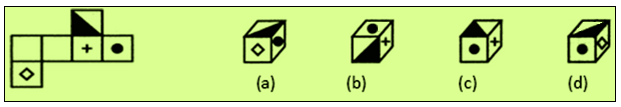a) a b) b c) c d) dSample PDF of SilverZone - International Reasoning & Aptitude Olympiad (iRAO) PDF Sample Papers for Class 6: